# Right Circular Cone Basics

Go back to  'Mensuration-Solids'

The following figure shows an example of a right circular cone: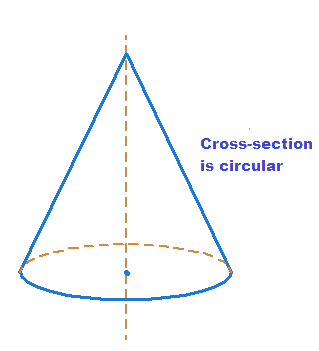Note that the cross section of this cone is circular. This means that if you cut the cone anywhere with a horizontal plane, you will get a circular cross-section: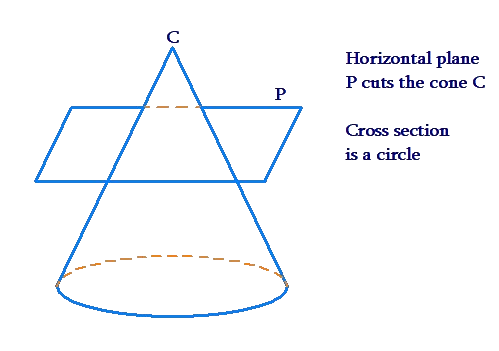Also, the axis of the cone is perpendicular to the base, and hence it is right: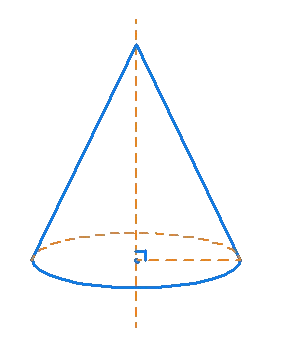The following figure shows a cone which is right but not circular (the cross-section is elliptical):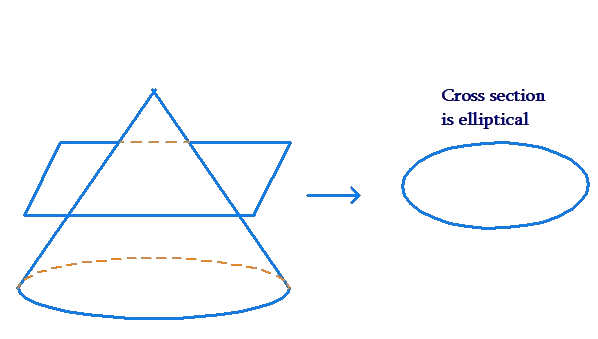The figure below shows a cone which is circular but not right (the axis is not perpendicular to the base):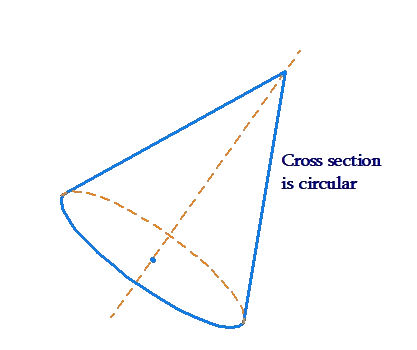From now on, we will discuss only right circular cones, and we will refer to them as simply cones. The dimensions of a cone can be specified by two parameters: the radius $$r$$of the base or the flat surface, and the height $$h$$ of the cone, as shown below.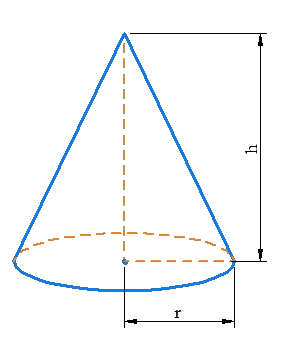## What is the formula for TSA of a circular cone?

• The formula for the total surface area of a right cone is T.S.A=πrl+πr^2 .

## What is a right circular cone?

• A right circular cone is a cone whose base is a circle and whose axis is perpendicular to the base. Such a cone can also be described as a solid formed by a right triangle rotated about one of its sides as an axis.

## What is the difference between right circular cone and cone?

• A right circular cone is a circular cone whose altitude intersects the plane of the circle at the circle's center. ... The only difference is the base--a pyramid is a cone with a polygonal base.

## How do you find the volume of a right circular cone?

• The volume V of a cone with radius r is one-third the area of the base B times the height h

## How do you find the radius of a right circular cone?

• A radius is the distance from the circle's middle to its perimeter, which is known as its circumference. The radius of a cone is the radius of its circular base. You can find a radius through its volume and height. Multiply the volume by 3.
Mensuration and Solids
Mensuration and Solids
grade 10 | Questions Set 2
Mensuration and Solids
Mensuration and Solids
grade 10 | Questions Set 1